You are using an outdated browser. Please upgrade your browser to improve your experience.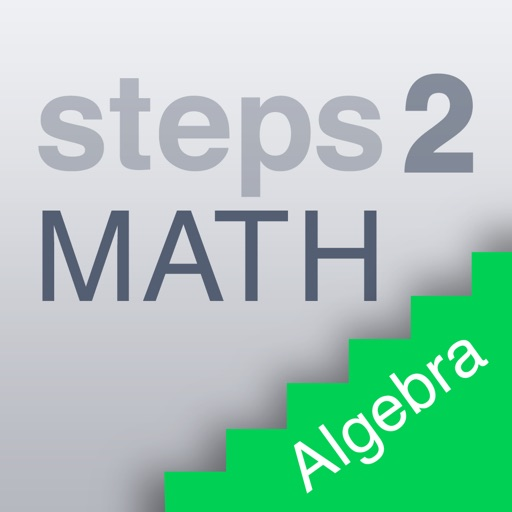No more "stupid math" and welcome to steps2Math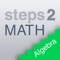steps2MATH

by Nees Consult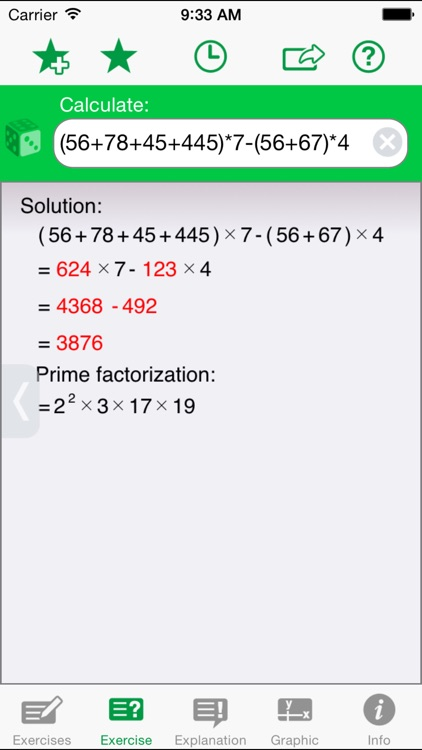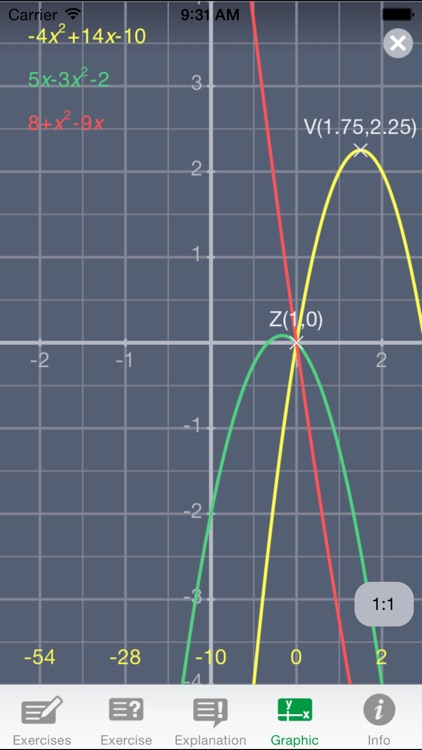What is it about?

No more "stupid math" and welcome to steps2Math. Our motto: "Understanding rather than despair." The math app gives students, parents, teachers from grades 5-11 easy access to mathematics. steps2Math solves not only math tasks. It shows and explains the individual steps in the corresponding solutions.App Details

Version
2.0.0
Rating
(11)
Size
14Mb
Genre
Education Productivity
Last updated
May 23, 2018
Release date
April 27, 2012

steps2MATH is \$0.99 but there are more add-ons

• \$11.99

all-inclusive

• \$4.99

• \$3.99

Exercises Level II

• \$1.99

Exercises Level III

• \$3.99

Support of measuring units

• \$3.99

Exercises Level I

App Screenshots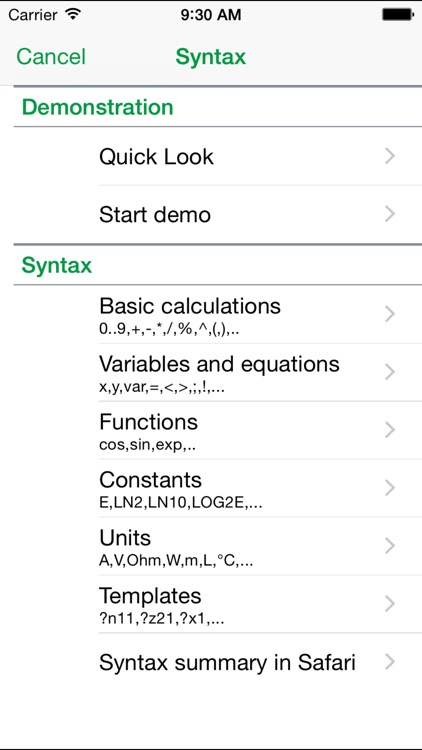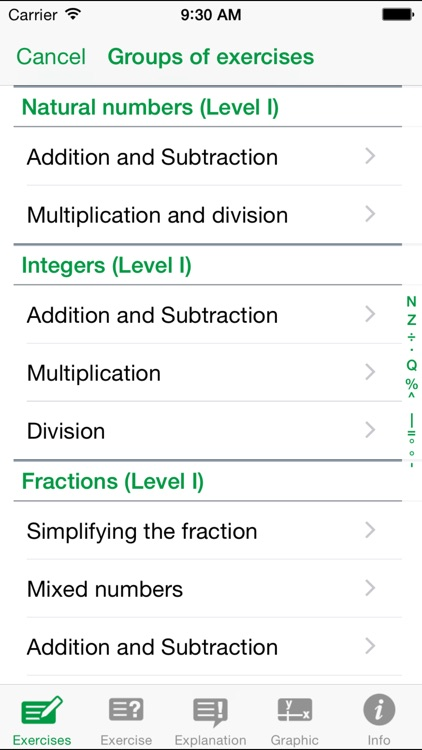App Store Description

No more "stupid math" and welcome to steps2Math. Our motto: "Understanding rather than despair." The math app gives students, parents, teachers from grades 5-11 easy access to mathematics. steps2Math solves not only math tasks. It shows and explains the individual steps in the corresponding solutions.

The functions of Steps2MATH:
• Algebra exercises can be entered.
• The solution is generated and displayed.
• For each transformation, there is an explanation.
• The transformations are shown in red and linked to the explanations.
• auxiliary calculation(s) (Addition, Division, Multiplication)
• Function graphs.
• Graphs for both sides of an equation.
• The graphics output includes a table of values.
• The graphs can be scaled and moved.
• For equations, the proof is carried out.
• 35 languages ​​are supported
• They can be switched at any time in the application.
• In some languages​​, the numbers can be displayed in the local representation.
• The application can be adapted to the needs and capabilities of the user.
• An extensive collection of exercises with more than 500 templates.
• The exercises are created again and again.
• Therefore Steps2MATH includes countless exercises.
• Calculations with exact numbers.
• Rounded values, if necessary.
• The exercises can be stored as documents.
• History
• Conversion and transformation of measurement units
• SI units: ampere ... Weber.
• Other units such as inches and yards.

The app can also be used as a scientific calculator.

Mathematical functions:
• Natural numbers
• Integers
• Fractions
• Decimal numbers
• Rational Numbers
• Percentage
• Exponentiation
• forming operations
• Linear equation
• Systems of linear equations
• Roots